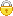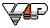﻿# A STUDY ON THE WEIGHT FUNCTION OF THE MOVING LEAST SQUARE APPROXIMATION IN THE LOCAL BOUNDARY INTEGRAL EQUATION METHOD

《固体力学学报：英文版》2003年 第3期 | LongShuyao HuDe‘an   DepartmentofEngineeringMechanics HunanUniversity Changsha410082 China论文服务： 摘　要：The meshless method is a new numerical technique presented in recent years .It uses the moving least square (MLS) approximation as a shape function . The smoothness of the MLS approximation is determined by that of the basic function and of the weight function, and is mainly determined by that of the weight function. Therefore, the weight function greatly affects the accuracy of results obtained. Different kinds of weight functions, such as the spline function, the Gauss function and so on, are proposed recently by many researchers. In the present work, the features of various weight functions are illustrated through solving elasto-static problems using the local boundary integral equation method. The effect of various weight functions on the accuracy, convergence and stability of results obtained is also discussed. Examples show that the weight function proposed by Zhou Weiyuan and Gauss and the quartic spline weight function are better than the others if parameters c and a in Gauss and exponential weight functions are in the range of reasonable values, respectively, and the higher the smoothness of the weight function, the better the features of the solutions.
 【分　类】 【数理科学和化学】 > 数学 > 数学分析 > 微分方程、积分方程 > 积分方程【数理科学和化学】 > 计算数学 > 数值分析 > 数值逼近 【关键词】 加权函数 网格方法 局部边界积分方程 移动最小二乘法 【出　处】 《固体力学学报：英文版》2003年 第3期 276-282页　共7页 【收　录】 中文科技期刊数据库